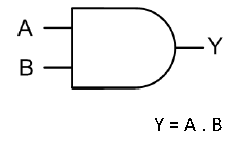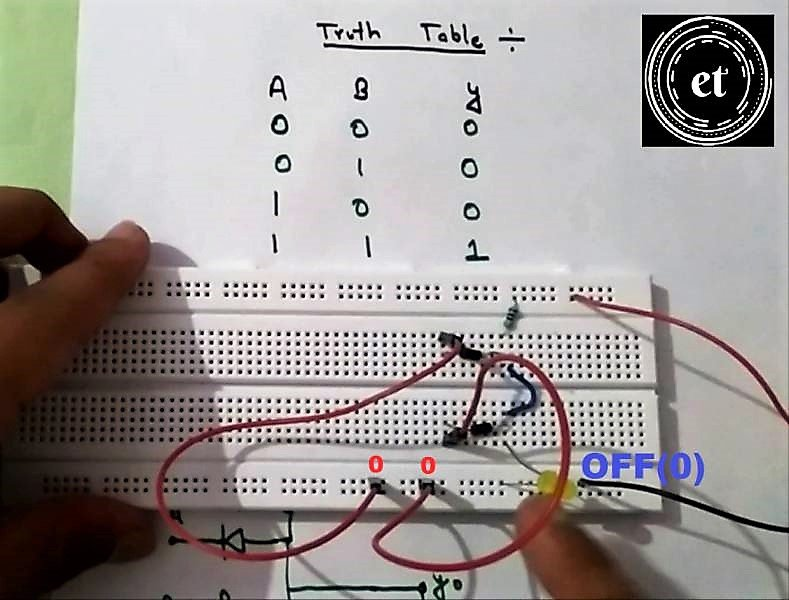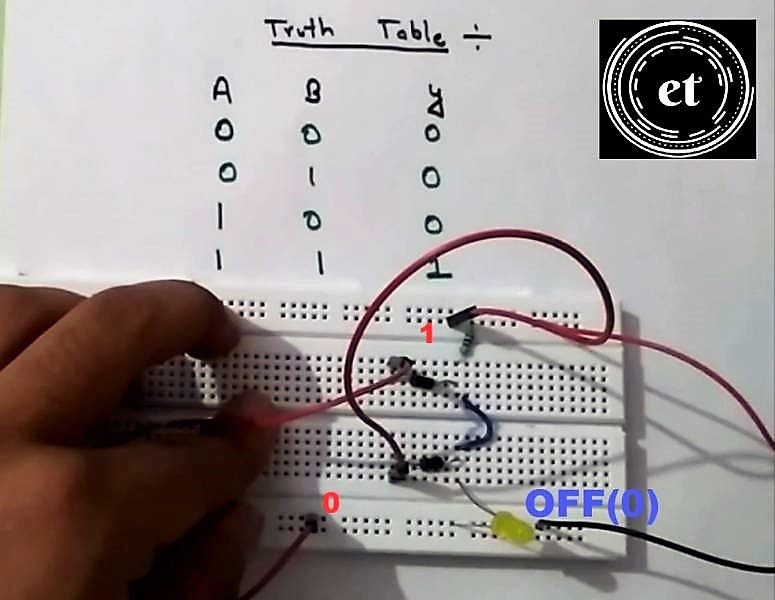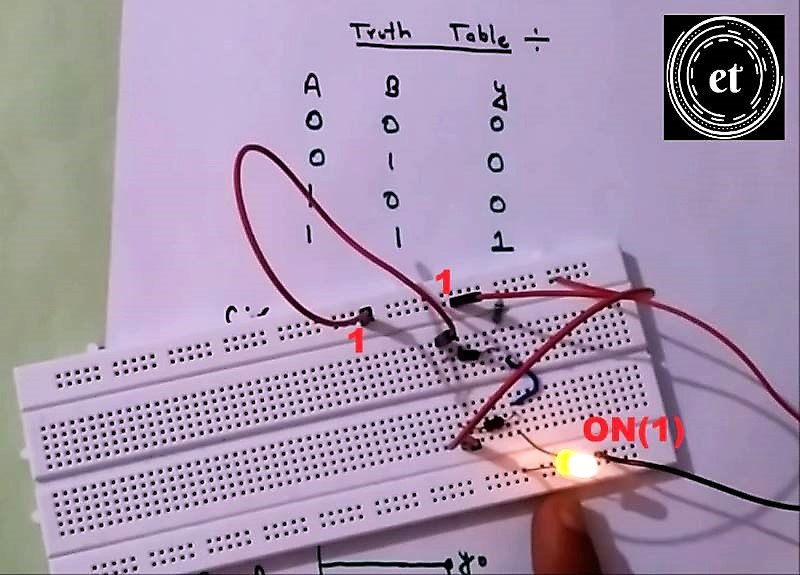# AND Gate using Diodes on Breadboard | AND Gate in detail

I am sure you must be familiar with an AND Gate, its Truth Table, Logic symbol, and its working. But do you know how to build your own And Gate on Breadboard? If not then don’t worry, we have got you covered. Because in this post, you will learn how to make an AND Gate using Diodes on Breadboard.

## AND Gate symbol and definition:AND gate is a simple three-terminal Logic Gate. It is called AND gate because AND means “to multiply”. There are two inputs and one output in an AND Gate. When both the inputs of the AND gate is HIGH, the output is HIGH. Whereas the output is LOW or Zero in all the other cases. The AND gate using diodes follow the same working principle.

## AND GATE Truth Table:

### Components required:

1. 1N4007 Diode X 2

2. 1k Resistor

3. LED

4. 9 Volt Battery

5. Connecting Wires

## Circuit Diagram of AND Gate using Diodes:

This is the circuit we are going to build on Breadboard.

1. P terminal of the diodes are connected together.

2. Logic inputs are given to n terminal of diodes.

3. End terminals of the Resistor are connected to P- terminals of the diode and +ve of 9 v battery respectively.

4. Output indicator i.e LED is connected from the P-terminal of the Diode to the Ground.

5. Ground is connected to the negative terminal of the battery.

## AND Gate working using Truth table:

NOTE: When the P-terminal of a Diode is at a higher potential (>.7v) then its n terminal, the diode acts as a short circuit. And when the n terminal is at a higher potential, the Diode act as an open circuit.

**from circuit diagram

CASE 1: Input A=0, Input B= 0, Output=0 (LED is OFF)

In this case, the p-terminal of both diodes is at a higher potential then n terminal and hence acts as a short circuit. Thus all current flow from the battery through 1k resistor, then diode to the Ground. Hence there is no voltage across LED and it remains off i.e, 0 at the output.

CASE 2: Input A=0, Input B= 1, Output=0 (LED is OFF)

In this case, the p-terminal of diode A is at a higher potential then n terminal. Hence the diode A acts as a short circuit. Thus all current flow from the battery through 1k resistor, then diode A to the Ground. So there is no voltage across LED and it remains off i.e, 0 at the output.

CASE 3: Input A=1, Input B= 0, Output=0 (LED is OFF)

In this case, the p-terminal of the diode B is at a higher potential than its n terminal and hence acts as a short circuit. Thus all current flow from the battery through 1k resistor, then diode B to the Ground. Hence there is no voltage across LED and it remains off i.e, 0 at the output.

CASE 4: Input A=1, Input B= 1, Output=1 (LED is ON)

In this case, the p-terminal of both diodes is at lower potential then n terminal. Hence both act as an open circuit. Thus, current flows from the battery through the 1k resistor to the Ground. Lighting up the LED on its way. So there is some voltage across LED and it is turned ON i.e, 1 at the output.

** Hence we get 1(LED is on) at the output only when both the inputs are 1 as given in Truth Table.

## AND Gate using diodes Circuit on Breadboard

1. Place the two diodes as shown below. N-terminal is the silver portion, P-terminal is the Black portion.

2. Connect two jumper wires at the N-terminal of each diode. The input logic to the gate is given through these wires. Call them A and B.

3. Connect the P terminal of each diode together.

4. Connect the resistor from p terminal of Diodes to V(battery’s +ve terminal)5. Connect the Battery’s +ve and -ve terminal to the breadboard as shown below.

6. Now connect an LED from P-terminals to the Gnd(battery’s -ve terminal).CASE 1: Input A=0, Input B= 0 , Output=0 (LED is OFF)In this case, the p-terminal of the diode B is at a higher potential than its n terminal and hence acts as a short circuit. Thus all current flow from the battery through 1k resistor, then diode B to the Ground. Hence there is no voltage across LED and it remains off i.e, 0 at the output.

CASE 2: Input A=0, Input B= 1, Output=0 (LED is OFF)In this case, the p-terminal of diode A is at a higher potential then n terminal. Hence the diode A acts as a short circuit. Thus all current flow from the battery through 1k resistor, then diode A to the Ground. So there is no voltage across LED and it remains off i.e, 0 at the output.

CASE 3: Input A=1, Input B= 0, Output=0 (LED is OFF)In this case, the p-terminal of the diode B is at a higher potential than its n terminal and hence acts as a short circuit. Thus all current flow from the battery through 1k resistor, then diode B to the Ground. Hence there is no voltage across LED and it remains off i.e, 0 at the output.

CASE 4: Input A=1, Input B= 1, Output=1 (LED is ON)In this case, the p-terminal of both diodes is at lower potential then n terminal. Hence both act as an open circuit. Thus, current flows from the battery through the 1k resistor to the Ground. Lighting up the LED on its way. So there is some voltage across LED and it is turned ON i.e, 1 at the output.

** Hence we get 1(LED is on) at the output only when both the inputs are 1 as given in Truth Table.

For the step by step video tutorial watch the video given below: